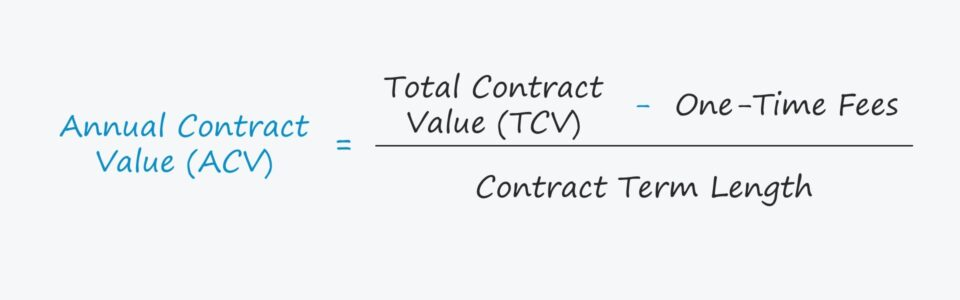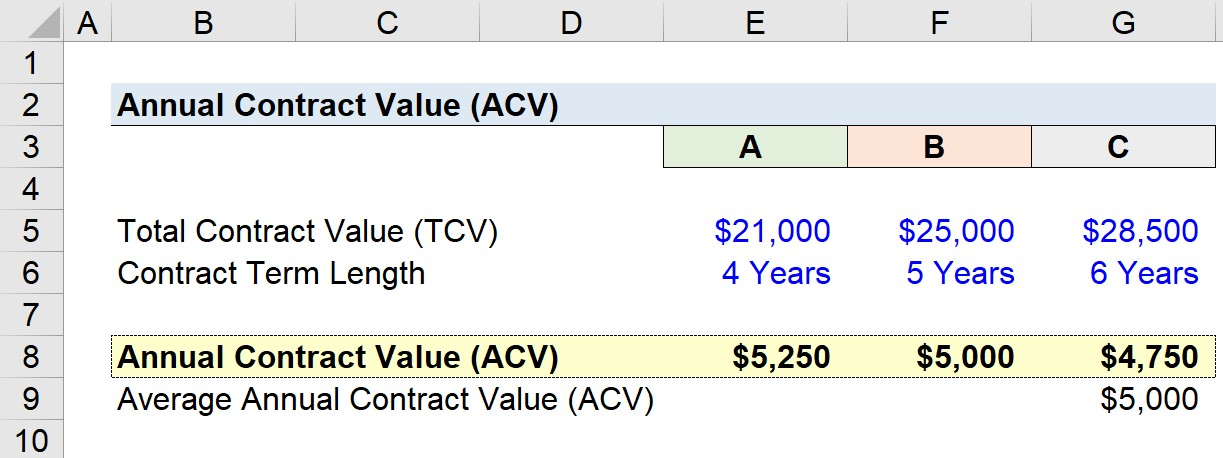Welcome to Wall Street Prep! Use code at checkout for 15% off.# Annual Contract Value (ACV)

Guide to Understanding Annual Contract Value (ACV)## How to Calculate Annual Contract Value (ACV)?

The annual contract value (ACV) is a KPI generally used to measure the ordinary revenue from a single, subscription-based customer contract.

SaaS and subscription-based companies operate business models oriented around generating recurring revenue. One method to obtain greater recurring revenue is through multi-year customer contracts, which represent commitments backed by a contractual obligation.

Once a customer signs a multi-year contract, the source of recurring revenue is close to “guaranteed” – barring unusual circumstances, e.g. if the customer undergoes bankruptcy, the customer decides to breach the contract in spite of the penalty, etc.

ACV is used to measure the average annualized revenue amount from a contract, whereas the TCV represents the entire revenue attributable to a contract.

## Annual Contract Value Formula (ACV)

The formula to calculate annual contract value (ACV) is calculated by dividing the normalized total contract value (TCV) and dividing by the contract term length.

“Normalized” in this context means that one-time fees are removed.

Annual Contract Value (ACV) = Normalized Total Contract Value (TCV) ÷ Contract Term Length

## What is the Difference Between ACV vs. TCV?

There are two common metrics for quantifying the revenue from customer contracts:

1. Total Contract Value (TCV): The total amount of revenue associated with a customer contract, inclusive of one-time fees.
2. Annual Contract Value (ACV): The annualized revenue expected from the average customer, excluding any one-time fees.

TCV is the total value of the customer contract and is thus independent of the time frame, i.e. the value does not change whether it is a one-year or ten-year contract.

But for the ACV, the value is directly affected by the contract duration, making it more useful for comparisons across the industry because it is an annualized metric.

Compared to TCV, the ACV metric can also be viewed as being more focused on recurring revenue since one-time fees such as onboarding and cancellation fees are NOT included.

While the total contract value (TCV) reflects the entire value of a new customer across the stated contract term length, the annual contract value (ACV) portrays only one year’s worth of revenue generated from the customer.

For example, let’s say that a customer signed a 4-year contract for \$40,000.

In this case, the total contract value (TCV) is \$40,000 whereas the annual contract value (ACV) is \$10,000.

• ACV = \$40,000 / 4 Years = \$10,000

## Annual Contract Value Calculator (ACV)

We’ll now move to a modeling exercise, which you can access by filling out the form below.Submitting...

## Step 1. SaaS Operating Assumptions

Suppose a SaaS startup has three customers, which we’ll refer to as customers A, B, and C.

The total contract value (TCV) of each customer and the contract length are listed below.

Customer A

• TCV = \$21,000
• Contract Term Length = 4 Years

Customer B

• TCV = \$25,000
• Contract Term Length = 5 Years

Customer C

• TCV = \$28,500
• Contract Term Length = 6 Years

## Step 2. Annual Contract Value Calculation Example (ACV)

In our simple example, the annual contract value (ACV) can be calculated individually and then added together to calculate the total ACV of all the contracts.

It is important to note that each customer holds only one contract. The ACV is \$5,250, \$5,000, and \$4,750 from customer A to C, respectively.

Customer A

• ACV = \$21,000 / 4 Years = \$5,250

Customer B

• ACV = \$25,000 / 5 Years = \$5,000

Customer C

• ACV = \$28,500 / 6 Years = \$4,750

The longer the contract duration, the lower the ACV to convince customers to agree to long-term contracts.

If we add together all three ACV values, the sum is \$15,000. And since there are three customer contracts, we can divide them by three to arrive at a total average annual contract value (ACV) of \$5,000.

• Average Annual Contract Value (ACV) = (\$5,250 + \$5,000 + \$4,750) / 3 Customers Contracts
• Average ACV = \$5,000Step-by-Step Online Course

### Everything You Need To Master Financial Modeling

Enroll in The Premium Package: Learn Financial Statement Modeling, DCF, M&A, LBO and Comps. The same training program used at top investment banks.Inline FeedbacksLearn Financial Modeling Online

Everything you need to master financial and valuation modeling: 3-Statement Modeling, DCF, Comps, M&A and LBO.

X

The Wall Street Prep Quicklesson Series

7 Free Financial Modeling Lessons

Get instant access to video lessons taught by experienced investment bankers. Learn financial statement modeling, DCF, M&A, LBO, Comps and Excel shortcuts.